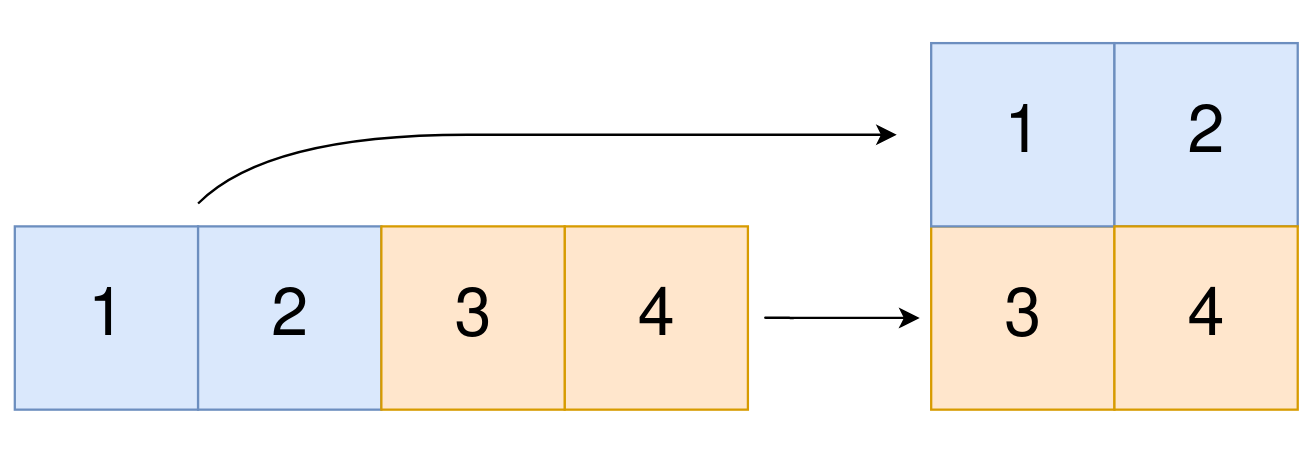## C# || How To Convert 1D Array Into 2D Array Using C#The following is a module with functions which demonstrates how to convert a 1D array into a 2D array using C#.

1. Construct 2D Array – Problem Statement

You are given a 0-indexed 1-dimensional (1D) integer array original, and two integers, m and n. You are tasked with creating a 2-dimensional (2D) array with m rows and n columns using all the elements from original.

The elements from indices 0 to n – 1 (inclusive) of original should form the first row of the constructed 2D array, the elements from indices n to 2 * n – 1 (inclusive) should form the second row of the constructed 2D array, and so on.

Return an m x n 2D array constructed according to the above procedure, or an empty 2D array if it is impossible.

Example 1:``` Input: original = [1,2,3,4], m = 2, n = 2 Output: [[1,2],[3,4]] Explanation: The constructed 2D array should contain 2 rows and 2 columns. The first group of n=2 elements in original, [1,2], becomes the first row in the constructed 2D array. The second group of n=2 elements in original, [3,4], becomes the second row in the constructed 2D array. ```

Example 2:

``` Input: original = [1,2,3], m = 1, n = 3 Output: [[1,2,3]] Explanation: The constructed 2D array should contain 1 row and 3 columns. Put all three elements in original into the first row of the constructed 2D array. ```

Example 3:

``` Input: original = [1,2], m = 1, n = 1 Output: [] Explanation: There are 2 elements in original. It is impossible to fit 2 elements in a 1x1 2D array, so return an empty 2D array. ```

Example 4:

``` Input: original = , m = 1, n = 2 Output: [] Explanation: There is 1 element in original. It is impossible to make 1 element fill all the spots in a 1x2 2D array, so return an empty 2D array. ```

2. Construct 2D Array – Solution

The following is a solution which demonstrates how to convert a 1D array into a 2D array.

``` 2. Construct 2D Array - Solution C# // ============================================================================ // Author: Kenneth Perkins // Date: Oct 18, 2021 // Taken From: http://programmingnotes.org/ // File: Solution.cs // Description: Demonstrates how to convert a 1D array into a 2D array // ============================================================================ public class Solution { public int[][] Construct2DArray(int[] original, int m, int n) { // Determine if parameters are valid if (original.Length != m * n) { return new int[]; } // Create 2D array var result = new int[m][]; for (int row = 0; row < result.Length; ++row) { result[row] = new int[n]; } // Populate result for (int index = 0; index < original.Length; ++index) { // Determine the result array row and column index var rowIndex = index / n; var colIndex = index % n; // Copy values to result result[rowIndex][colIndex] = original[index]; } return result; } }// http://programmingnotes.org/ 123456789101112131415161718192021222324252627282930313233 // ============================================================================//    Author: Kenneth Perkins//    Date:   Oct 18, 2021//    Taken From: http://programmingnotes.org///    File:  Solution.cs//    Description: Demonstrates how to convert a 1D array into a 2D array// ============================================================================public class Solution {    public int[][] Construct2DArray(int[] original, int m, int n) {        // Determine if parameters are valid        if (original.Length != m * n) {            return new int[];        }         // Create 2D array        var result = new int[m][];        for (int row = 0; row < result.Length; ++row) {            result[row] = new int[n];        }         // Populate result        for (int index = 0; index < original.Length; ++index) {            // Determine the result array row and column index            var rowIndex = index / n;            var colIndex = index % n;             // Copy values to result            result[rowIndex][colIndex] = original[index];        }         return result;    }}// http://programmingnotes.org/ ```

QUICK NOTES:
The highlighted lines are sections of interest to look out for.

The code is heavily commented, so no further insight is necessary. If you have any questions, feel free to leave a comment below.

Once compiled, you should get this as your output for the example cases:

``` [[1,2],[3,4]] [[1,2,3]] [] [] ```